• ## 非参数估计：核密度估计KDE

万次阅读 多人点赞 2016-12-14 11:38:46
http://blog.csdn.net/pipisorry/article/details/53635895核密度估计Kernel Density Estimation(KDE)概述密度估计的问题...参数估计参数估计可分为参数回归分析和参数判别分析。在参数回归分析中，人们假定数据分布
http://blog.csdn.net/pipisorry/article/details/53635895核密度估计Kernel Density Estimation(KDE)概述密度估计的问题由给定样本集合求解随机变量的分布密度函数问题是概率统计学的基本问题之一。解决这一问题的方法包括参数估计和非参数估计。参数估计参数估计又可分为参数回归分析和参数判别分析。在参数回归分析中，人们假定数据分布符合某种特定的性态，如线性、可化线性或指数性态等，然后在目标函数族中寻找特定的解，即确定回归模型中的未知参数。在参数判别分析中，人们需要假定作为判别依据的、随机取值的数据样本在各个可能的类别中都服从特定的分布。经验和理论说明，参数模型的这种基本假定与实际的物理模型之间常常存在较大的差距，这些方法并非总能取得令人满意的结果。[参数估计：最大似然估计MLE][参数估计：文本分析的参数估计方法]非参数估计方法由于上述缺陷，Rosenblatt和Parzen提出了非参数估计方法，即核密度估计方法。由于核密度估计方法不利用有关数据分布的先验知识，对数据分布不附加任何假定，是一种从数据样本本身出发研究数据分布特征的方法，因而，在统计学理论和应用领域均受到高度的重视。核密度估计（kernel density estimation）是在概率论中用来估计未知的密度函数，属于非参数检验方法之一，由Rosenblatt (1955)和Emanuel Parzen(1962)提出，又名Parzen窗（Parzen window）。Ruppert和Cline基于数据集密度函数聚类算法提出修订的核密度估计方法。核密度估计在估计边界区域的时候会出现边界效应。[https://zh.wikipedia.org/zh-hans/核密度估计]因此，一句话概括，核密度估计Kernel Density Estimation(KDE)是在概率论中用来估计未知的密度函数，属于非参数检验方法之一。在密度函数估计中有一种方法是被广泛应用的——直方图。如下图中的第一和第二幅图（名为Histogram和Histogram, bins shifted）。直方图的特点是简单易懂，但缺点在于以下三个方面：密度函数是不平滑的；密度函数受子区间（即每个直方体）宽度影响很大，同样的原始数据如果取不同的子区间范围，那么展示的结果可能是完全不同的。如下图中的前两个图，第二个图只是在第一个图的基础上，划分区间增加了0.75，但展现出的密度函数却看起来差异很大；直方图最多只能展示2维数据，如果维度更多则无法有效展示。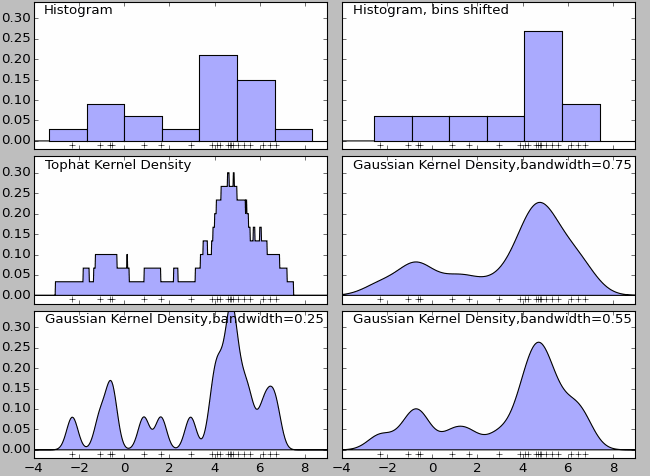核密度估计有多种内核，图3（Tophat Kernl Density）为不平滑内核，图4（Gaussian Kernel Density,bandwidth=0.75）为平滑内核。在很多情况下，平滑内核（如高斯核密度估计，Gaussian Kernel Density）使用场景较多。虽然采用不同的核函数都可以获得一致性的结论（整体趋势和密度分布规律性基本一致），但核密度函数也不是完美的。除了核算法的选择外，带宽（bandwidth）也会影响密度估计，过大或过小的带宽值都会影响估计结果。如上图中的最后三个图，名为Gaussian Kernel Density,bandwidth=0.75、Gaussian Kernel Density,bandwidth=0.25、Gaussian Kernel Density,bandwidth=0.55.核密度估计的应用场景股票、金融等风险预测：在单变量核密度估计的基础上，可以建立风险价值的预测模型。通过对核密度估计变异系数的加权处理，可以建立不同的风险价值的预测模型。密度估计中应用较多的算法是高斯混合模型以及基于近邻的核密度估计。高斯混合核密度估计模型更多会在聚类场景中应用。[核密度估计Kernel Density Estimation(KDE)]核密度分析可用于测量建筑密度、获取犯罪情况报告，以及发现对城镇或野生动物栖息地造成影响的道路或公共设施管线。可使用 population 字段根据要素的重要程度赋予某些要素比其他要素更大的权重，该字段还允许使用一个点表示多个观察对象。例如，一个地址可以表示一栋六单元的公寓，或者在确定总体犯罪率时可赋予某些罪行比其他罪行更大的权重。对于线要素，分车道高速公路可能比狭窄的土路产生更大的影响，高压线要比标准电线杆产生更大的影响。[ArcGIS中的介绍]热力图大家一定听说过，其实热力图就是核密度估计。总而言之，核密度就是用来估计密度的，如果你有一系列空间点数据，那么核密度估计往往是比较好的可视化方法皮皮blog核密度估计所谓核密度估计，就是采用平滑的峰值函数(“核”)来拟合观察到的数据点，从而对真实的概率分布曲线进行模拟。核密度估计（Kernel density estimation），是一种用于估计概率密度函数的非参数方法，为独立同分布F的n个样本点，设其概率密度函数为f，核密度估计为以下：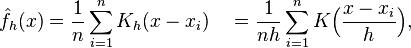K(.)为核函数（非负、积分为1，符合概率密度性质，并且均值为0）。有很多种核函数，uniform,triangular, biweight, triweight, Epanechnikov,normal等。h>0为一个平滑参数，称作带宽(bandwidth)，也看到有人叫窗口。Kh(x) = 1/h K(x/h). 为缩放核函数(scaled Kernel)。核密度函数的原理比较简单，在我们知道某一事物的概率分布的情况下，如果某一个数在观察中出现了，我们可以认为这个数的概率密度很大，和这个数比较近的数的概率密度也会比较大，而那些离这个数远的数的概率密度会比较小。基于这种想法，针对观察中的第一个数，我们可以用K去拟合我们想象中的那个远小近大概率密度。对每一个观察数拟合出的多个概率密度分布函数，取平均。如果某些数是比较重要的，则可以取加权平均。需要说明的一点是，核密度的估计并不是找到真正的分布函数。Note: 核密度估计其实就是通过核函数（如高斯）将每个数据点的数据+带宽当作核函数的参数，得到N个核函数，再线性叠加就形成了核密度的估计函数，归一化后就是核密度概率密度函数了。以下面3个数据点的一维数据集为例：5, 10, 15绘制成直方图是这样的：                                                   而使用KDE则是：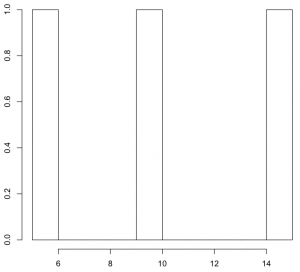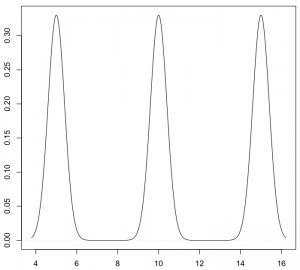KDE核函数k(.)理论上，所有平滑的峰值函数均可作为KDE的核函数来使用，只要对归一化后的KDE而言(描绘在图上的是数据点出现的概率值)，该函数曲线下方的面积和等于1即可。 只有一个数据点时，单个波峰下方的面积为1，存在多个数据点时，所有波峰下方的面积之和为1。概而言之，函数曲线需囊括所有可能出现的数据值的情况。常用的核函数有：矩形、Epanechnikov曲线、高斯曲线等。这些函数存在共同的特点：在数据点处为波峰；曲线下方面积为1。单个数据点（只有一个数据时）所对应的这些核函数矩形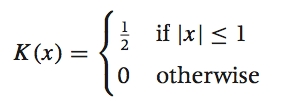Epanechnikov曲线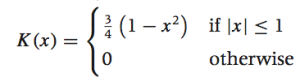高斯曲线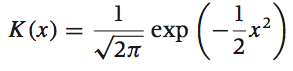[概率论：高斯/正态分布                    ]sklearn中实现的核函数sklearn核函数形式Gaussian kernel (kernel = 'gaussian')Tophat kernel (kernel = 'tophat')ifEpanechnikov kernel (kernel = 'epanechnikov')Exponential kernel (kernel = 'exponential')Linear kernel (kernel = 'linear')ifCosine kernel (kernel = 'cosine')if[Kernel Density Estimation¶]wekipedia上各种核函数的图形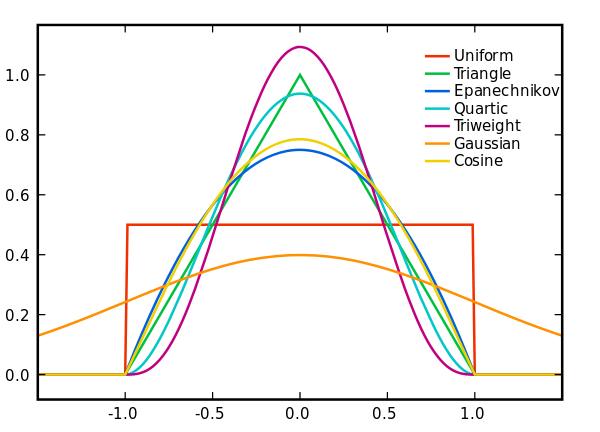均匀核函数 k(x)=1/2,-1≤x≤1 加入带宽h后： kh(x)=1/(2h),-h≤x≤h三角核函数 k(x)=1-|x|,-1≤x≤1 加入带宽h后： kh(x)=(h-|x|)/h^2,-h≤x≤h伽马核函数 kxi(x)=[x^(α-1)exp{-xα/xi}]/[(xi/α)^α.Γ(α)]高斯核函数K(x,xc)=exp(-||x-xc||^2/(2*σ)^2)其中xc为核函数中心,σ为函数的宽度参数[https://zh.wikipedia.org/zh-hans/%E6%A0%B8%E5%AF%86%E5%BA%A6%E4%BC%B0%E8%AE%A1]不同内核的比较 Epanechnikov 内核在均方误差意义下是最优的，效率损失也很小。由于高斯内核方便的数学性质，也经常使用 K(x)= ϕ(x)，ϕ(x)为标准正态概率密度函数。对于多个数据点的KDE曲线：由于相邻波峰之间会发生波形合成，因此最终所形成的曲线形状与选择的核函数关系并不密切。考虑到函数在波形合成计算上的易用性，一般使用高斯曲线(正态分布曲线)作为KDE的核函数。KDE算法：索引树lz发现sklearn算法实现中有一个参数是算法项，如algorithm='auto'，想了一下是为了加速。KDE的概率密度函数公式得到后有了上述公式之后，只需遍历输出图像的每一个点，计算其核密度估计值即可。但是稍微想一下就发现这个程序太冗余了，如果有很多点（n很大），并且输出图像很大，那么每一个像素都需要进行n个累积的加法运算，并且大部分都是+0（因为一般来说，一个点附近的点不会很多，远远小于n，其余大部分点与这个像素的距离都大于r），这样就造成了冗余计算。解决方案当然也非常简单，就是建立一个索引，然后在计算某个像素的核密度估计值时利用索引搜索出附近的点，然后累积这些点的核函数即可。如Dotspatial自带了多种空间索引，有R树，R*树，KD树等；sklearn自带了kd tree, ball tree等等。如果只需找出附近的点，对索引要求不高，任意一个索引都能使用。[空间点云核密度估计算法的实现-以Dotspatial为基础GIS库]KDE带宽h如何选定核函数的“方差”呢？这其实是由带宽h来决定，不同的带宽下的核函数估计结果差异很大。带宽反映了KDE曲线整体的平坦程度，也即观察到的数据点在KDE曲线形成过程中所占的比重。带宽越大，观察到的数据点在最终形成的曲线形状中所占比重越小，KDE整体曲线就越平坦；带宽越小，观察到的数据点在最终形成的曲线形状中所占比重越大，KDE整体曲线就越陡峭。还是以上面3个数据点的一维数据集为例，如果增加带宽，那么生成的KDE曲线就会变平坦：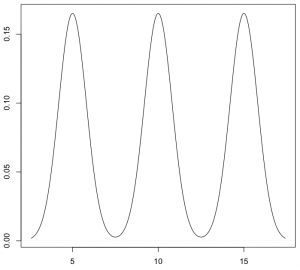如果进一步增加带宽，那么KDE曲线在变平坦的同时，还会发生波形合成：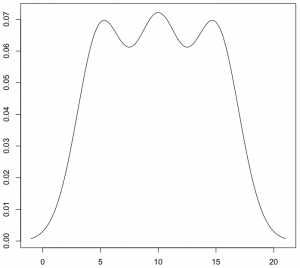相反，如果减少带宽，那么KDE曲线就会变得更加陡峭：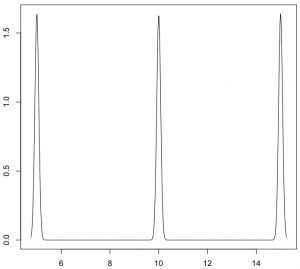从数学上来说，对于数据点Xi，如果带宽为h，那么在Xi处所形成的曲线函数为(其中K为核函数)：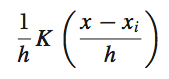在上面的函数中，K函数内部的h分母用于调整KDE曲线的宽幅，而K函数外部的h分母则用于保证曲线下方的面积符合KDE的规则(KDE曲线下方面积和为1)。带宽的选择带宽的选择很大程度上取决于主观判断：如果认为真实的概率分布曲线是比较平坦的，那么就选择较大的带宽；相反，如果认为真实的概率分布曲线是比较陡峭的，那么就选择较小的带宽。带宽计算好像也有相应的方法，如R语言中计算带宽时，默认采用”nrd0″方法。如何选择h？显然是选择可以使误差最小的。下面用平均积分平方误差（mean intergrated squared error）的大小来衡量h的优劣。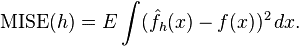在weak assumptions下，MISE (h) =AMISE(h) + o(1/(nh) + h4) ，其中AMISE为渐进的MISE。而AMISE有，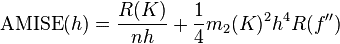，其中，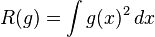，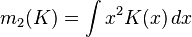为了使MISE(h)最小，则转化为求极点问题，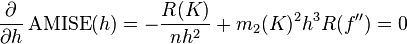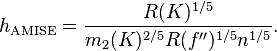当核函数确定之后，h公式里的R、m、f''都可以确定下来，有（hAMISE ~ n−1/5），AMISE(h) = O(n−4/5)。        如果带宽不是固定的，其变化取决于估计的位置（balloon  estimator）或样本点（逐点估计pointwise estimator），由此可以产产生一个非常强大的方法称为自适应或可变带宽核密度估计。[核密度估计(Kernel density estimation)                    ]在选择合适的核函数及带宽后，KDE可以模拟真实的概率分布曲线，并得到平滑而漂亮的结果。以近200个点的CPU使用率为例，使用KDE绘制的结果为：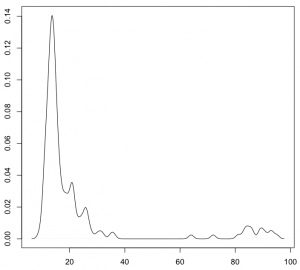[一维数据可视化：核密度估计(Kernel Density Estimates)]皮皮blog核密度估计的实现Python中KDE的实现：sklearn[sklearn.neighbors.KernelDensity(bandwidth=1.0, algorithm='auto', kernel='gaussian', metric='euclidean', atol=0, rtol=0, breadth_first=True, leaf_size=40, metric_params=None)from sklearn.neighbors import kde
import numpy as np

X = np.array([[-1, -1], [-2, -1], [-3, -2], [1, 1], [2, 1], [3, 2]])
kde = kde.KernelDensity(kernel='gaussian', bandwidth=0.2).fit(X)
print(kde.score_samples(X))
print(np.exp(kde.score_samples(X)))[-0.41075698 -0.41075698 -0.41076071 -0.41075698 -0.41075698 -0.41076071][ 0.66314807  0.66314807  0.6631456   0.66314807  0.66314807  0.6631456 ]score_samples(X)Evaluate the density model on the data.Parameters:X : array_like, shape (n_samples, n_features)kde.score_samples(X)返回的是点x对应概率的log值，要使用exp求指数还原。Note: 还原后的所有点的概率和范围是[0, 无穷大]，只是说一维数据线下面的面积或者二维数据面下面的体积和为1。[Density Estimation¶][sklearn.neighbors.KernelDensity¶]spark中KDE的实现MLlib中，仅仅支持以高斯核做核密度估计。[核密度估计]R中KDE的实现在R语言中，KDE的绘制是通过density()函数来实现的 — 通过density()函数计算得到KDE模型，然后再使用plot()函数对KDE曲线进行绘制：x <- c(5, 10, 15)plot(density(x))出于兼容性上的考虑，R语言中density()函数在计算带宽时，默认采用”nrd0″方法。不过，根据R语言的帮助文档，带宽参数bw应该显式声明为其它更合适的方法，比如”SJ”：plot(density(x, bw="SJ"))对于调整带宽，除了修改bw参数，还可以通过设定adjust参数来进行扩大或缩小：plot(density(x, bw="SJ", adjust=1.5))在上面的例子中，最终使用的带宽将是采用”SJ”方法计算得到的带宽的1.5倍。adjust参数的默认值为1，也即既不扩大、也不缩小。至于核函数，density()默认采用高斯曲线。可以通过设定kernel参数来更改核函数。比如：plot(density(x, bw="SJ", kernel="epanechnikov"))density()函数接受以下7个核函数选项：    gaussian。高斯曲线，默认选项。在数据点处模拟正态分布。    epanechnikov。Epanechnikov曲线。    rectangular。矩形核函数。    triangular。三角形核函数。    biweight。    cosine。余弦曲线。    optcosine。from: http://blog.csdn.net/pipisorry/article/details/53635895ref: [有边界区间上的核密度估计]
展开全文kde 核密度估计
• 参数估计参数估计可分为参数回归分析和参数判别分析。在参数回归分析中，人们假定数据分布符合某种特定的性态，如线性、可化线性或指数性态等，然后在目标函数族中寻找特定的解，即确定回归模型中的未知参数。...
核密度估计Kernel Density Estimation(KDE)概述密度估计的问题由给定样本集合求解随机变量的分布密度函数问题是概率统计学的基本问题之一。解决这一问题的方法包括参数估计和非参数估计。参数估计参数估计又可分为参数回归分析和参数判别分析。在参数回归分析中，人们假定数据分布符合某种特定的性态，如线性、可化线性或指数性态等，然后在目标函数族中寻找特定的解，即确定回归模型中的未知参数。在参数判别分析中，人们需要假定作为判别依据的、随机取值的数据样本在各个可能的类别中都服从特定的分布。经验和理论说明，参数模型的这种基本假定与实际的物理模型之间常常存在较大的差距，这些方法并非总能取得令人满意的结果。非参数估计方法由于上述缺陷，Rosenblatt和Parzen提出了非参数估计方法，即核密度估计方法。由于核密度估计方法不利用有关数据分布的先验知识，对数据分布不附加任何假定，是一种从数据样本本身出发研究数据分布特征的方法，因而，在统计学理论和应用领域均受到高度的重视。核密度估计(kernel density estimation)是在概率论中用来估计未知的密度函数，属于非参数检验方法之一，由Rosenblatt (1955)和Emanuel Parzen(1962)提出，又名Parzen窗(Parzen window)。Ruppert和Cline基于数据集密度函数聚类算法提出修订的核密度估计方法。核密度估计在估计边界区域的时候会出现边界效应。[https://zh.wikipedia.org/zh-hans/核密度估计]因此，一句话概括，核密度估计Kernel Density Estimation(KDE)是在概率论中用来估计未知的密度函数，属于非参数检验方法之一。在密度函数估计中有一种方法是被广泛应用的——直方图。如下图中的第一和第二幅图(名为Histogram和Histogram, bins shifted)。直方图的特点是简单易懂，但缺点在于以下三个方面：密度函数是不平滑的；密度函数受子区间(即每个直方体)宽度影响很大，同样的原始数据如果取不同的子区间范围，那么展示的结果可能是完全不同的。如下图中的前两个图，第二个图只是在第一个图的基础上，划分区间增加了0.75，但展现出的密度函数却看起来差异很大；直方图最多只能展示2维数据，如果维度更多则无法有效展示。核密度估计有多种内核，图3(Tophat Kernl Density)为不平滑内核，图4(Gaussian Kernel Density,bandwidth=0.75)为平滑内核。在很多情况下，平滑内核(如高斯核密度估计，Gaussian Kernel Density)使用场景较多。虽然采用不同的核函数都可以获得一致性的结论(整体趋势和密度分布规律性基本一致)，但核密度函数也不是完美的。除了核算法的选择外，带宽(bandwidth)也会影响密度估计，过大或过小的带宽值都会影响估计结果。如上图中的最后三个图，名为Gaussian Kernel Density,bandwidth=0.75、Gaussian Kernel Density,bandwidth=0.25、Gaussian Kernel Density,bandwidth=0.55.核密度估计的应用场景股票、金融等风险预测：在单变量核密度估计的基础上，可以建立风险价值的预测模型。通过对核密度估计变异系数的加权处理，可以建立不同的风险价值的预测模型。密度估计中应用较多的算法是高斯混合模型以及基于近邻的核密度估计。高斯混合核密度估计模型更多会在聚类场景中应用。[核密度估计Kernel Density Estimation(KDE)]核密度分析可用于测量建筑密度、获取犯罪情况报告，以及发现对城镇或野生动物栖息地造成影响的道路或公共设施管线。可使用 population 字段根据要素的重要程度赋予某些要素比其他要素更大的权重，该字段还允许使用一个点表示多个观察对象。例如，一个地址可以表示一栋六单元的公寓，或者在确定总体犯罪率时可赋予某些罪行比其他罪行更大的权重。对于线要素，分车道高速公路可能比狭窄的土路产生更大的影响，高压线要比标准电线杆产生更大的影响。[ArcGIS中的介绍]热力图大家一定听说过，其实热力图就是核密度估计。总而言之，核密度就是用来估计密度的，如果你有一系列空间点数据，那么核密度估计往往是比较好的可视化方法核密度估计所谓核密度估计，就是采用平滑的峰值函数(“核”)来拟合观察到的数据点，从而对真实的概率分布曲线进行模拟。核密度估计(Kernel density estimation)，是一种用于估计概率密度函数的非参数方法，为独立同分布F的n个样本点，设其概率密度函数为f，核密度估计为以下：h>0为一个平滑参数，称作带宽(bandwidth)，也看到有人叫窗口。Kh(x) = 1/h K(x/h). 为缩放核函数(scaled Kernel)。核密度函数的原理比较简单，在我们知道某一事物的概率分布的情况下，如果某一个数在观察中出现了，我们可以认为这个数的概率密度很大，和这个数比较近的数的概率密度也会比较大，而那些离这个数远的数的概率密度会比较小。基于这种想法，针对观察中的第一个数，我们可以用K去拟合我们想象中的那个远小近大概率密度。对每一个观察数拟合出的多个概率密度分布函数，取平均。如果某些数是比较重要的，则可以取加权平均。需要说明的一点是，核密度的估计并不是找到真正的分布函数。Note: 核密度估计其实就是通过核函数(如高斯)将每个数据点的数据+带宽当作核函数的参数，得到N个核函数，再线性叠加就形成了核密度的估计函数，归一化后就是核密度概率密度函数了。以下面3个数据点的一维数据集为例：5, 10, 15绘制成直方图是这样的：                                                   而使用KDE则是：KDE核函数k(.)理论上，所有平滑的峰值函数均可作为KDE的核函数来使用，只要对归一化后的KDE而言(描绘在图上的是数据点出现的概率值)，该函数曲线下方的面积和等于1即可。只有一个数据点时，单个波峰下方的面积为1，存在多个数据点时，所有波峰下方的面积之和为1。概而言之，函数曲线需囊括所有可能出现的数据值的情况。常用的核函数有：矩形、Epanechnikov曲线、高斯曲线等。这些函数存在共同的特点：在数据点处为波峰；曲线下方面积为1。单个数据点(只有一个数据时)所对应的这些核函数矩形Epanechnikov曲线高斯曲线sklearn中实现的核函数sklearn核函数形式Gaussian kernel (kernel = 'gaussian')Tophat kernel (kernel = 'tophat')ifEpanechnikov kernel (kernel = 'epanechnikov')Exponential kernel (kernel = 'exponential')Linear kernel (kernel = 'linear')ifCosine kernel (kernel = 'cosine')if[Kernel Density Estimation¶]wekipedia上各种核函数的图形均匀核函数 k(x)=1/2,-1≤x≤1 加入带宽h后： kh(x)=1/(2h),-h≤x≤h三角核函数 k(x)=1-|x|,-1≤x≤1 加入带宽h后： kh(x)=(h-|x|)/h^2,-h≤x≤h伽马核函数 kxi(x)=[x^(α-1)exp{-xα/xi}]/[(xi/α)^α.Γ(α)]高斯核函数K(x,xc)=exp(-||x-xc||^2/(2*σ)^2)其中xc为核函数中心,σ为函数的宽度参数[https://zh.wikipedia.org/zh-hans/%E6%A0%B8%E5%AF%86%E5%BA%A6%E4%BC%B0%E8%AE%A1]不同内核的比较Epanechnikov 内核在均方误差意义下是最优的，效率损失也很小。由于高斯内核方便的数学性质，也经常使用 K(x)= ϕ(x)，ϕ(x)为标准正态概率密度函数。对于多个数据点的KDE曲线：由于相邻波峰之间会发生波形合成，因此最终所形成的曲线形状与选择的核函数关系并不密切。考虑到函数在波形合成计算上的易用性，一般使用高斯曲线(正态分布曲线)作为KDE的核函数。KDE算法：索引树lz发现sklearn算法实现中有一个参数是算法项，如algorithm='auto'，想了一下是为了加速。KDE的概率密度函数公式得到后有了上述公式之后，只需遍历输出图像的每一个点，计算其核密度估计值即可。但是稍微想一下就发现这个程序太冗余了，如果有很多点(n很大)，并且输出图像很大，那么每一个像素都需要进行n个累积的加法运算，并且大部分都是+0(因为一般来说，一个点附近的点不会很多，远远小于n，其余大部分点与这个像素的距离都大于r)，这样就造成了冗余计算。解决方案当然也非常简单，就是建立一个索引，然后在计算某个像素的核密度估计值时利用索引搜索出附近的点，然后累积这些点的核函数即可。如Dotspatial自带了多种空间索引，有R树，R*树，KD树等；sklearn自带了kd tree, ball tree等等。如果只需找出附近的点，对索引要求不高，任意一个索引都能使用。[空间点云核密度估计算法的实现-以Dotspatial为基础GIS库]KDE带宽h如何选定核函数的“方差”呢？这其实是由带宽h来决定，不同的带宽下的核函数估计结果差异很大。带宽反映了KDE曲线整体的平坦程度，也即观察到的数据点在KDE曲线形成过程中所占的比重。带宽越大，观察到的数据点在最终形成的曲线形状中所占比重越小，KDE整体曲线就越平坦；带宽越小，观察到的数据点在最终形成的曲线形状中所占比重越大，KDE整体曲线就越陡峭。还是以上面3个数据点的一维数据集为例，如果增加带宽，那么生成的KDE曲线就会变平坦：如果进一步增加带宽，那么KDE曲线在变平坦的同时，还会发生波形合成：相反，如果减少带宽，那么KDE曲线就会变得更加陡峭：从数学上来说，对于数据点Xi，如果带宽为h，那么在Xi处所形成的曲线函数为(其中K为核函数)：在上面的函数中，K函数内部的h分母用于调整KDE曲线的宽幅，而K函数外部的h分母则用于保证曲线下方的面积符合KDE的规则(KDE曲线下方面积和为1)。带宽的选择带宽的选择很大程度上取决于主观判断：如果认为真实的概率分布曲线是比较平坦的，那么就选择较大的带宽；相反，如果认为真实的概率分布曲线是比较陡峭的，那么就选择较小的带宽。带宽计算好像也有相应的方法，如R语言中计算带宽时，默认采用”nrd0″方法。如何选择h？显然是选择可以使误差最小的。下面用平均积分平方误差(mean intergrated squared error)的大小来衡量h的优劣。在weak assumptions下，MISE (h) =AMISE(h) + o(1/(nh) + h4) ，其中AMISE为渐进的MISE。而AMISE有，，其中，，为了使MISE(h)最小，则转化为求极点问题，当核函数确定之后，h公式里的R、m、f''都可以确定下来，有(hAMISE ~ n−1/5)，AMISE(h) = O(n−4/5)。如果带宽不是固定的，其变化取决于估计的位置(balloon  estimator)或样本点(逐点估计pointwise estimator)，由此可以产产生一个非常强大的方法称为自适应或可变带宽核密度估计。[核密度估计(Kernel density estimation)]在选择合适的核函数及带宽后，KDE可以模拟真实的概率分布曲线，并得到平滑而漂亮的结果。以近200个点的CPU使用率为例，使用KDE绘制的结果为：[一维数据可视化：核密度估计(Kernel Density Estimates)]核密度估计的实现Python中KDE的实现：sklearn[sklearn.neighbors.KernelDensity(bandwidth=1.0, algorithm='auto', kernel='gaussian', metric='euclidean', atol=0, rtol=0, breadth_first=True, leaf_size=40, metric_params=None)from sklearn.neighbors import kdeimport numpy as npX = np.array([[-1, -1], [-2, -1], [-3, -2], [1, 1], [2, 1], [3, 2]])kde = kde.KernelDensity(kernel='gaussian', bandwidth=0.2).fit(X)print(kde.score_samples(X))print(np.exp(kde.score_samples(X)))[-0.41075698 -0.41075698 -0.41076071 -0.41075698 -0.41075698 -0.41076071][ 0.66314807  0.66314807  0.6631456   0.66314807  0.66314807  0.6631456 ]score_samples(X)Evaluate the density model on the data.Parameters:X : array_like, shape (n_samples, n_features)kde.score_samples(X)返回的是点x对应概率的log值，要使用exp求指数还原。Note: 还原后的所有点的概率和范围是[0, 无穷大]，只是说一维数据线下面的面积或者二维数据面下面的体积和为1。[Density Estimation¶][sklearn.neighbors.KernelDensity¶]spark中KDE的实现MLlib中，仅仅支持以高斯核做核密度估计。R中KDE的实现在R语言中，KDE的绘制是通过density()函数来实现的 — 通过density()函数计算得到KDE模型，然后再使用plot()函数对KDE曲线进行绘制：x plot(density(x))出于兼容性上的考虑，R语言中density()函数在计算带宽时，默认采用”nrd0″方法。不过，根据R语言的帮助文档，带宽参数bw应该显式声明为其它更合适的方法，比如”SJ”：plot(density(x, bw="SJ"))对于调整带宽，除了修改bw参数，还可以通过设定adjust参数来进行扩大或缩小：plot(density(x, bw="SJ", adjust=1.5))在上面的例子中，最终使用的带宽将是采用”SJ”方法计算得到的带宽的1.5倍。adjust参数的默认值为1，也即既不扩大、也不缩小。至于核函数，density()默认采用高斯曲线。可以通过设定kernel参数来更改核函数。比如：plot(density(x, bw="SJ", kernel="epanechnikov"))density()函数接受以下7个核函数选项：gaussian。高斯曲线，默认选项。在数据点处模拟正态分布。epanechnikov。Epanechnikov曲线。rectangular。矩形核函数。triangular。三角形核函数。biweight。cosine。余弦曲线。optcosine。
展开全文• 参数估计参数估计非随机时常参数估计最大似然估计（Maximum Likelihood Estimator）最小二乘估计（Least Squares Estimator）最大...根据待估计参数随时间变化与否可分为时常参数和时变参数，其中对时常参数的估计称为
参数估计参数估计非随机时常参数估计最大似然估计（Maximum Likelihood Estimator）最小二乘估计（Least Squares Estimator）最大似然估计和最小二乘估计对比随机时常参数估计最大后验概率估计（Maximum A Posteriori Estimator）最小均方误差估计（Minimum Mean Square Error Estimator）最大后验概率估计与最小均方误差估计对比
参数估计
根据待估计参数随时间变化与否可分为时常参数和时变参数，其中对时常参数的估计称为参数估计，对时变参数的估计称为状态估计，这里我们研究的是时常参数估计。对时常参数$x$的估计问题可以表述为，已知观测$z(j)=h[j,x,\omega(j)],~~~j=1,2,...,k$其中，$\omega(j)$为噪声干扰，用已观测集合$Z^k=\{z(1),z(2),...,z(k)\}$进行估计，得到估计量为$\hat{x}(k):=\hat{x}[k,Z^k]$估计的误差表示为$\tilde{x}:=x-\hat{x}$时常参数估计可以根据待估计量的随机性分为两大种类：非随机时常参数估计，随机时常参数估计。

非随机时常参数估计容易理解，参数是确定的未知常量，也被叫做非贝叶斯方法（Non-Bayesian Approach）
随机时常参数是指参数是个随机变量，参数取值遵循一定分布$p(x)$但参数不随时间变化，也被称作贝叶斯方法（Bayesian Approach）

非随机时常参数估计
最大似然估计（Maximum Likelihood Estimator）
先观察一个概率，假定参数为$x$条件下得到那组观测集合的概率为$\Lambda_k(x):=p(Z^k|x)$参数的改变会使得观测也随之发生改变，$x$是因而$Z^k$是果。在得到观测$Z^k$结果时，反观是谁最可能导致这一现象的发生。这是最大似然估计的主要思想，似然也是可能的意思，$\Lambda_k(x)$被称为似然函数。最大似然估计过程为：找到一个$x$使得概率$p(Z^k|x)$最大，数学描述为$\hat{x}=\text{arg} ~\text{max} ~p(Z|x)$最大似然估计的解可以通过令似然函数求导等于零求得$\dfrac{\text{d}p(Z|x)}{\text{d}x}=0$不难看出，我们需要知道这一条件分布$p(Z|x)$，也就是，需要知道噪声分布的先验信息。
最小二乘估计（Least Squares Estimator）
另外一种处理非随机时常参数估计的方法是最小二乘估计，它所选取的最优准则为：最优估计$\hat{x}$可以使所有量测的误差平方和总体达到最小，也就是$\hat{x}=\text{arg} ~\text{min} ~\left\{\sum_{j=1}^k[z(j)-h(j,x)]^2\right\}$这里需要注意，最小二乘估计对噪声无任何假设条件，无需知道噪声的分布特性。有关更多最小二乘估计内容参见之前的笔记 最小二乘估计（Least squares estimation）
最大似然估计和最小二乘估计对比
最小二乘估计不依赖于有关噪声的先验分布信息，而最大似然估计需要已知条件概率分布
为了充分对比，假定最小二乘估计中噪声的分布也是已知的，且与最大似然估计中噪声分布一致，同为均值为0方差为$\sigma^2$的高斯噪声$\omega_j\sim \mathcal{N}(0,\sigma^2)$观测的分布为$z(j)\sim \mathcal{N}(h(j,x),\sigma^2)$似然函数为$\Lambda_k(x)=p(Z^k|x)=p[z(1),z(2),...,z(k)|x]$因为观测之间相互独立，等于各自条件分布的乘积\begin{aligned}\Lambda_k(x)&= \prod_{j=1}^k\mathcal{N}(z(j);h(j,x),\sigma^2)\\&=c~\text{exp}\left({-\frac{1}{2\sigma^2}\sum_{j=1}^k}[z(j)-h(j,x)]^2\right)\end{aligned}可以看到使似然函数最大，也就是使指数项最小。这时候，最大似然估计等价于最小二乘估计。
随机时常参数估计
随机时常参数的估计也被称作贝叶斯估计，贝叶斯估计的准则为：使平均代价最小
估计的误差为$x-\hat{x}$，人们要为这种误差付出代价，代价用代价函数表示$c(x,\hat{x})$，常用的代价函数有

均匀代价函数
估计值在真值附近一个范围$\Delta$内是不付出任何代价的，其余的代价满足均匀分布，即
$c(x,\hat{x})=\begin{cases} 1, & |x-\hat{x}|\ge\frac{\Delta}{2} \\ 0, & |x-\hat{x}|<\frac{\Delta}{2} \end{cases}$
平方代价函数
误差的平方作为代价函数，即
$c(x,\hat{x})=(x-\hat{x})^2$

平均代价可以由代价函数和先验分布函数得到\begin{aligned}\bar{c}&=\int\int c(x,\hat{x})p(x,Z)\text{d}x\text{d}Z\\&=\int\int c(x,\hat{x})p(x|Z)p(Z)\text{d}x\text{d}Z\\&=\int\left[\int c(x,\hat{x})p(x|Z)\text{d}x\right]p(Z)\text{d}Z=\int\bar{c}(\hat{x}|Z)p(Z)\text{d}Z\end{aligned}使平均代价最小也就是使条件平均代价$\bar{c}(\hat{x}|Z)$最小
最大后验概率估计（Maximum A Posteriori Estimator）
后验概率的理解为，结果作为已知去寻求发生的原因，听起来和最大似然估计很像对不对，不妨带着疑问接着往下看。采取均匀代价函数，计算条件平均代价为\begin{aligned}\bar{c}(\hat{x}|Z)&=\int c(x,\hat{x})p(x|Z)\text{d}x\\&=\int_{-\infty}^{\hat{x}-\frac{\Delta}{2}}p(x|Z)\text{d}x+\int_{\hat{x}+\frac{\Delta}{2}}^{\infty}p(x|Z)\text{d}x\\&=1-\int_{\hat{x}-\frac{\Delta}{2}}^{\hat{x}+\frac{\Delta}{2}}p(x|Z)\text{d}x\end{aligned}
当$\Delta\to0$时，$p(x|Z)$越大则条件平均代价越小，实则使后验概率最大，数学描述为$\hat{x}=\text{arg} ~\text{max} ~p(x|Z)=\text{arg} ~\text{max} ~[p(Z|x)p(x)]$上式用到了贝叶斯定理并且估计值和分母上的常数无关。相较于最大似然估计，这里多了一项$x$的先验概率$p(x)$。
最大后验概率估计比最大似然估计有更多的先验信息，从这方面来看，最大后验概率估计要比最大似然估计准确的多，前提是先验信息是准确的。
最小均方误差估计（Minimum Mean Square Error Estimator）
采取平方代价函数，条件平均代价为
\begin{aligned}\bar{c}(\hat{x}|Z)&=\int c(x,\hat{x})p(x|Z)\text{d}x\\&=\int_{-\infty}^{\infty}(x-\hat{x})^2p(x|Z)\text{d}x\end{aligned}最小均方误差估计便是找到使均方误差$E[(x-\hat{x})^2|Z]=\int_{-\infty}^{\infty}(x-\hat{x})^2p(x|Z)\text{d}x$最小的$x$值$\hat{x}=\text{arg} ~\text{min} ~E[(x-\hat{x})^2|Z]$对均方误差求一阶导数为\begin{aligned}\dfrac{\text{d}\bar{c}(\hat{x}|Z)}{\text{d}\hat{x}}&=\dfrac{\text{d}\int_{-\infty}^{\infty}(x-\hat{x})^2p(x|Z)\text{d}x}{\text{d}\hat{x}}\\&=-2\int_{-\infty}^{\infty}(x-\hat{x})p(x|Z)\text{d}x\\&=-2\int_{-\infty}^{\infty}xp(x|Z)\text{d}x+2\hat{x}\int_{-\infty}^{\infty}p(x|Z)\text{d}x\end{aligned}其二阶导数大于零，令一阶导数等于零求得$\hat{x}=\int_{-\infty}^{\infty}xp(x|Z)\text{d}x=E[x|Z]$最小均方误差的估计值为条件均值
最大后验概率估计与最小均方误差估计对比
假定参数$x$是具有均值$\bar{x}$、方差为$\sigma_x^2$的高斯随机变量，噪声$\omega$满足零均值，方差为$\sigma_\omega^2$的高斯分布。并且参数与噪声是不相关的$z=x+\omega$那么，量测的概率密度函数满足$p(z)=\mathcal{N}(z;\bar{x},\sigma_x^2+\sigma_\omega^2)$后验概率为$p(x|z)=\dfrac{1}{\sqrt{2\pi}\sigma}\text{exp}\left\{-\dfrac{[x-f(z)]^2}{2\sigma ^2}\right\}$其中$\sigma=\dfrac{\sigma_x^2 \sigma_\omega^2}{\sigma_x^2+ \sigma_\omega^2}~~~f(z)=\bar{x}+\dfrac{\sigma_x^2}{\sigma_x^2+ \sigma_\omega^2}(z-\bar{x})$最大后验概率估计值为$\hat{x}=f(z)$，$p(x|z)$在高斯分布下的条件均值也是$f(z)$
若$x$分布的方差为无限大，相当于无任何先验信息，最大后验估计的估计值为$z$，与最大似然估计结果一致


展开全文• ## 参数估计概念整理

千次阅读 2018-05-15 14:29:51
分为点估计和区间估计两个部分统计量：可以简单理解为根据样本构造的概率密度函数参数空间：参数估计中，我们假设总体的概率密度函数已知，而未知的是函数中的几个参数，用Θ表示。Θ的所有可能取值即为参数空间。...
参数估计：所谓参数估计即根据总体中抽取的样本估计总体分布中的未知参数。分为点估计和区间估计两个部分统计量：可以简单理解为根据样本构造的概率密度函数参数空间：参数估计中，我们假设总体的概率密度函数已知，而未知的是函数中的几个参数，用Θ表示。Θ的所有可能取值即为参数空间。贝叶斯估计：针对贝叶斯分类，通常我们知道先验概率p(w)和类条件概率p(x|w),根据贝叶斯公式可以求出后验概率p(w|x),据此可以对样本进行分类。然而很多情况下，我们只有一些样本，而先验概率及类条件概率也需要估计得出。如果训练样本的分布能够代表样本的真实分布，每个样本都是独立同分布iid，则根据样本类别的频度可以估计出先验概率p(w)。而针对类条件概率可以由估计概率密度p(x|w)转为估计参数，即根据样本和要求选择适当模型并进行参数估计。即模型已定参数未知。最大似然估计：利用已知的样本反推最有可能导致这样结果的参数值似然函数：针对样本集D={x1,x2,x3....xn}来估计参数向量Θ，概率密度函数p(D|Θ)成为相对于D的Θ的似然函数。l(Θ)=p(D|Θ)=∏(i=1 - i=n)p(xi|Θ)若Θhat是参数空间内使l(Θ)最大的参数，则其为最可能的参数值，称作极大似然函数估计值。Θhat为样本集的函数：Θhat=d(D)。
展开全文• 理解略肤浅，所以该博文更适合像我一样的初学者...根据对象是一个值，还是一个区间，可分为估计和区间估计 3、矩估计 首先我们应该了解什么是矩呢？这是一个数理统计概念： 中心矩，原点矩一个意思。 矩估计...
• 一、本章知识结构 参数估计是统计推断的最基本问题之一，分为点估计和区间估计。其研究任务是：总体的分布已知，但含有未知参数；我们要通过对样本进行加工，提取有用信息，对未知参数作出估计。本章要熟悉如何对...
• ## R语言中的参数估计

千次阅读 2020-12-25 11:52:44
以下我将从点估计、区间估计来介绍区间估计，本文主要介绍R代码，具体的统计知识，详情参考相关数理统计的专业书嗷参数估计R语言中的参数估计点估计距估计极大似然估计区间估计均值μ\muμ的估计两样本方差比的...算法
• 二是网络参数估计，即已知网络结构，估计每个条件概率分布的参数。 不含隐变量的参数估计 如果图模型中不包含隐变量，即所有变量都是观测的，那么网络参数一般可以直接通过最大似然来进行估计。 含隐变量的参数...
• 交通流的演化过程一般可分为交通流的量变与质变。鉴于交通自由流状态、拥挤流状态及间歇流状态的分布均可由伽马分布表达，利用伽马分布及其变点检验算法进行变点搜索及检验，确定变点前后的交通流状态。结合...
• 基音估计 基音是语音信号的重要参数，在语音产生的数字模型中，也是激励源的一个重要参数。在汉语中，基音的变化模式为声调，可以帮助区别意义。准确检测语音信号的基音周期对高质量的语音分析与合成、语音压缩编码...
• 其中，总体均值μ的区间估计可分为两种：方差^2已知时的均值的区间估计和方差^2未知时的均值的区间估计。(1)方差^2已知是的均值的区间估计在R中没有现成的求已知方差时均值的区间估计的函数，因此我们这里首先就是...
• 根据目标先验信息数据库建立的方式，合成孔径雷达(SAR)目标识别可分为基于模板的目标识别和基于模型的目标识别两类，模板数量的有限性限制了第1种方法的应用。采用合适的模型并调整模型参数预测目标在各种姿态及环境...
•人工智能 机器学习
• 转自：机器学习——极大似然估计与贝叶斯估计 极大似然估计 极大似然估计是频率派提出的参数的点估计方法。...可分为贝叶斯点估计，贝叶斯区间估计，本文暂不涉及区间估计。 基于参数theta是服从一定先验分...机器学习
• ## 核密度估计KDE

千次阅读 2018-08-19 11:28:49
核密度估计Kernel Density Estimation(KDE)概述 密度估计的问题 由给定样本集合求解随机变量的分布密度函数问题是概率统计学的基本问题之一。...参数估计可分为参数回归分析和参数判别分析。在参数回归分析...
• 参数估计可分为参数回归分析和参数判别分析。在参数回归分析中，人们假定数据分布符合某种特定的性态，如线性、可化线性或指数性态等，然后在目标函数族中寻找特定的解，即确定回归模型中的...
• 在介绍核密度评估Kernel Density Estimation(KDE)之前，先介绍下密度估计的问题。由给定样本集合求解随机变量的分布密度函数...参数估计可分为参数回归分析和参数判别分析。在参数回归分析中，人们假定数据分布...
•人工智能 机器学习
• 自适应滤波算法在功率谱估计中的应用研究，贾存丽，郭娜，功率谱估计是分析随机信号的一种重要分析方法，功率谱估计一般可分为经典谱估计(非参数估计)和现代谱估计(参数估计)两种方法，在��
• 回归模型经过各种检验并标明符合预定的要求后，...（1）平均值的点估计：实际上是对总体参数估计 是利用估计的回归方程，对于x的一个特定值，求出y的平均值的一个估计值 （2）个别值的点估计：对因变量的某个具...
• 运动估计计算法可分为两大类，即基于非参数运动模型的运动估计和基于参数模型的运动估计。非参数运动估计需要显式的边界条件才能获取物体的运动信息，比如光流场方程和随机场法。参数模型运动估计假设每一个图像块都...hevc
• 其内容极其丰富,涉及的学科和领域也相当广泛,按是否有参数大致可分为参数模型估计和非参数模型估计,前者有AR模型、MA模型、ARMA模型、PRONY指数模型等;后者有最小方差方法、多分量的MUSIC方法等。 ARMA谱估计叫做...
• 我们描述了具有重尾瑞利分布的多视极化SAR图像的成功建模，并提出了基于矩阵对数累积量的重尾瑞利分布包括等效视数（ENL）的新颖参数估计器。 首先，将重尾瑞利分布的复合变量分为正α稳定变量和复数高斯变量的乘积...
• 回顾VaR的定义，为未来收益的累计分布函数，那么 所以，VaR本质上为未来收益的分位点。要计算它，最重要的是估计未来收益的分布。...后一个方向成为模拟法，在实际使用中，又可分为历史模拟法和蒙特卡洛模拟法...
• 全书分为两卷，分别讲解了统计信号处理基础的估计理论和检测理论。 第一卷详细介绍了经典估计理论和贝叶斯估计，总结了各种估计方法，考虑了维纳滤波和卡尔曼滤波，并介绍了对复数据和参数估计方法。本卷给出了100...
• 说的是讲深度学习和图像的几何原理结合起来进行端到端的学习，分为两个阶段：运动估计和深度估计 1 介绍： 就是说从视频中求得深度图，传统的的方法是使用sfm,来求优化求得3D结构和相机的运动，然后将相机的参数...
• 对破碎过程最重要的因素分为三类：爆炸参数，岩石参数和爆炸几何形状。 岩石碎裂取决于两个主要因素，即不控制的岩石特性和可操纵以达到最大效率的爆破参数。 观察后记录选定的变数量，密度，装药长度，爆破量...
• 程序基于“Fast and reliable structure-oriented video noise estimation”并做了改进，分为针对CFA图像、灰度图像、RGB三个版本，各子通道方差综合方法可以修正，程序留出了第三个参数用于扩展。程序实现上可......